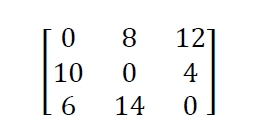# C++ Program to Implement Johnson’s Algorithm

Here we will see the Johnson’s Algorithm to find shortest path between two vertices.The graph is given here. The shortest path between the edges is like below. This program will take the number of vertices, number of edges, and the edges with their costs.

Input − Vertices: 3

Edges: 5

Edge with costs −

1 2 8

2 1 12

1 3 22

3 1 6

2 3 4

Output − The distance matrix of the graph.

 0 8 12 10 0 4 6 14 0

## Algorithm

### johnsonAlgorithm(cost)

Input − The cost matrix of given Graph.

Output − Matrix to for shortest path between any vertex to any vertex.

Begin
Create another matrix ‘A’ same as cost matrix, if there is no edge between ith row and jth column, put infinity at A[i,j].
for k := 1 to n, do
for i := 1 to n, do
for j := 1 to n, do
A[i, j] = minimum of A[i, j] and (A[i, k] + A[k, j])
done
done
done
display the current A matrix
End

## Example

Live Demo

#include<iostream>
#define INF 9999
using namespace std;
int min(int a, int b);
inline int min(int a, int b){
return (a<b)?a:b;
}
main() {
int vert, edge, i, j, k, c;
cout << "Enter no of vertices: ";
cin >> vert;
cout << "Enter no of edges: ";
cin >> edge;
cout << "Enter the EDGE Costs:\n";
for (k = 1; k <= edge; k++) { //take the input and store it into adj and cost matrix
cin >> i >> j >> c;
}
for (i = 1; i <= vert; i++)
for (j = 1; j <= vert; j++) {
if (adj[i][j] == 0 && i != j)
adj[i][j] = INF; //if there is no edge, put infinity
}
for (k = 1; k <= vert; k++)
for (i = 1; i <= vert; i++)
for (j = 1; j <= vert; j++)
for (i = 1; i <= vert; i++) {
for (j = 1; j <= vert; j++) {
cout << adj[i][j] << " ";
}
cout << "\n";
}
}

## Output

Enter no of vertices: 3
Enter no of edges: 5
Enter the EDGE Costs:
1 2 8
2 1 12
1 3 22
3 1 6
2 3 4
6 14 0